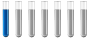Stoichiometry HelpAuthor Message

NewbieJoined: 14 Feb 2018
Posts: 3# Stoichiometry Help

1. If 8.6 L of H2 reacted with 4.3 L of O2 at STP, what is the theoretical volume of the gaseous water collected (assuming that none of it condenses)? 2H2(g) + O2(g) -> 2H2O

2. The decomposition of potassium chlorate yields oxygen gas. If the yield is 95%, how many grams of KClO3 are needed to produce 10 L of O2? 2KClO3(s) -> 2KCl(s) + 3O2(g)

3. Considered the following reaction: 2H2S(g) +3O2(g) -> 2SO2(g) + 2H2O(g)
If O2 was the excess reagent, 8.3 moles of H2S were consumed, and 137.1g of water were collected after the reaction has gone to completion, what is the percent yield of this reaction?

4. Consider the following reaction: 2H2S(g) +3O2(g) -> 2SO2(g) + 2H2O(g)
If 2L of O2 were provided, and 8.3 moles of H2S were available, which reactant is in excess and how much will remain after the reaction is complete?Distinguished MemberJoined: 27 Sep 2017
Posts: 264
Location: Berlin, GermanyFor all questions, convert the given amounts to mole. Then compare how much mole in the equation is needed and see what reactant is in excess. 1. 8,6 l H2 is 0,383 mol and 4,3 l O2 is 0,192 mol. Ratio is 2 to 1 same like in the equation. So you will get 0,383 mole H2O steam, what mean its 8,6 l The other try by yourself, if questions then asked.Display posts from previous: All Posts1 Day7 Days2 Weeks1 Month3 Months6 Months1 Year Oldest FirstNewest FirstPage 1 of 1

 Jump to: Select a forum Chemistry----------------Chemistry forum